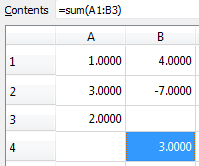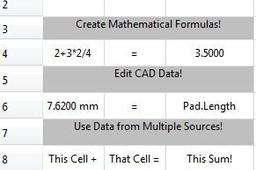1. ### Step 1: Setting up the Spreadsheet

To get started, go to the Spreadsheet workbench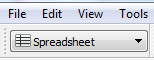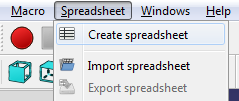Quick Tip: Workbench specific features (such as "Create spreadsheet") are also accessible from the ribbon bar.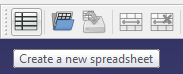A new spreadsheet will show up in the model tree. Double clicking on it will open the spreadsheet as a new tab, which shows up at the bottom of your screen.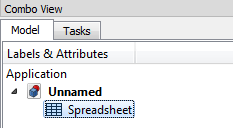It will open as another tab at the bottom of your screen, you can switch between the spreadsheet and model tabs anytime you want.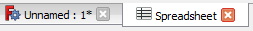The spreadsheet is auto saved so you can click the 'X' at any time without losing your work.

2. ### Step 2: Text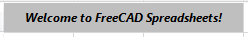Just start typing to add text to the selected cell. You can edit it at any time by clicking on the cell you want to edit and going up to the "Contents" or by pressing f2.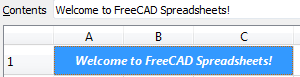You can edit colors, alignment, and text styling at any time by using the buttons on the ribbon bar above the spreadsheet, or by right clicking on the cell and clicking properties.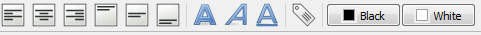3. ### Step 3: Math - Numbers and Formulas

Spreadsheets can also do math! Try inputting "2+2" into a cell, then press Enter. You can also do subtraction -  division /  multiplication * exponent ^

When putting a formula into FreeCAD, remember that multiplication and division will be calculated first regardless of the order that a formula is typed. To force a specific part of a formula to be done first, there must be parenthesis () around it.

Example: 1+2*3=7 but (1+2)*3=9

You can do other mathematical functions too such as: sum(), sqrt(), abs(), sin(), cos(), tan(), log(). For functions with a () after them, the value you want calculated must be INSIDE the parenthesis.

Example: sum(1+2)

Sometimes it's helpful to type a formula out without it getting calculated, to do this put an apostrophe ' at the beginning of the text.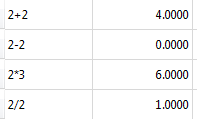Left column: text with an apostrophe ' in front of it. Right column: same text, but this time without the apostrophe '

Having issues? FreeCAD spreadsheets automatically add equals signs to the start of formulas but occasionally it doesn't work. If your formula doesn't auto calculate, try putting an = in front of it.

Example: =sum(2+3)

4. ### Step 4: Referencing Other Cells

Spreadsheets can also reference other cells! Type a number into two different cells, in this case I used A1 and B1. In a different cell type in: A1+B1 Click off the cell or press enter and your answer appear.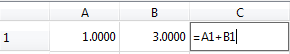A1 and B1 must have capitalized letters to work. a1 and b1 will not work.

Select a range of cells by putting a colon : between the starting and ending cell locations. In this example, the sum of 6 cells (A1 to B3) was calculated inside cell B4. Notice that positive and negative numbers, as well as filled in and blank cells are allowed.